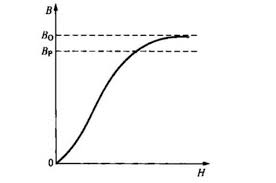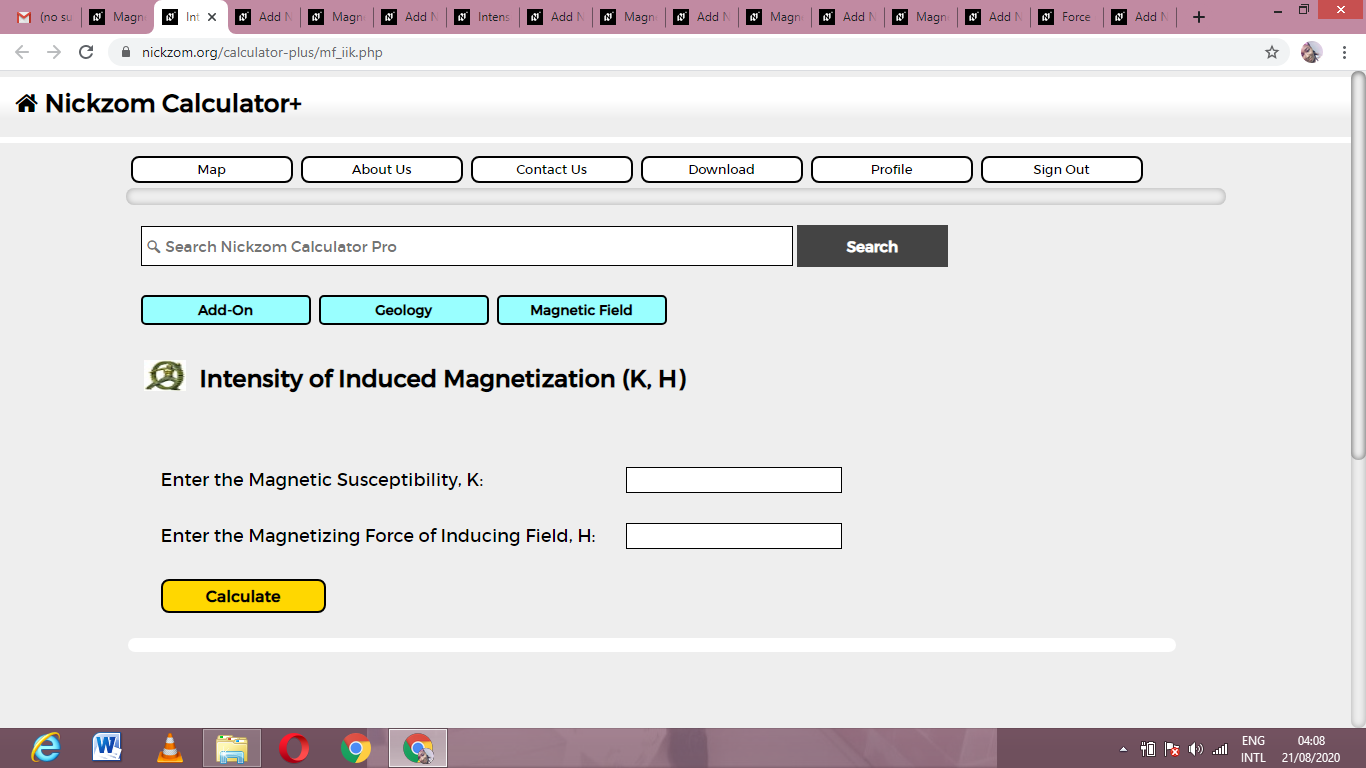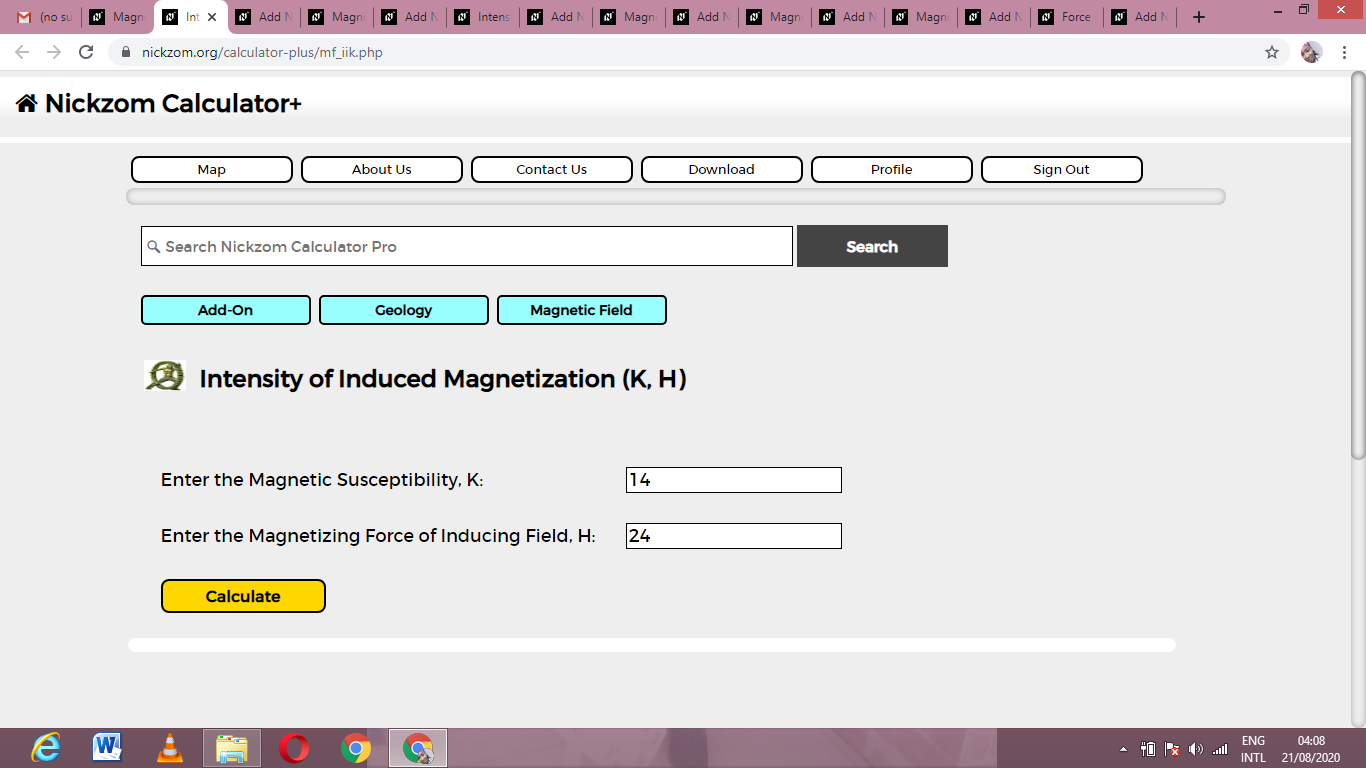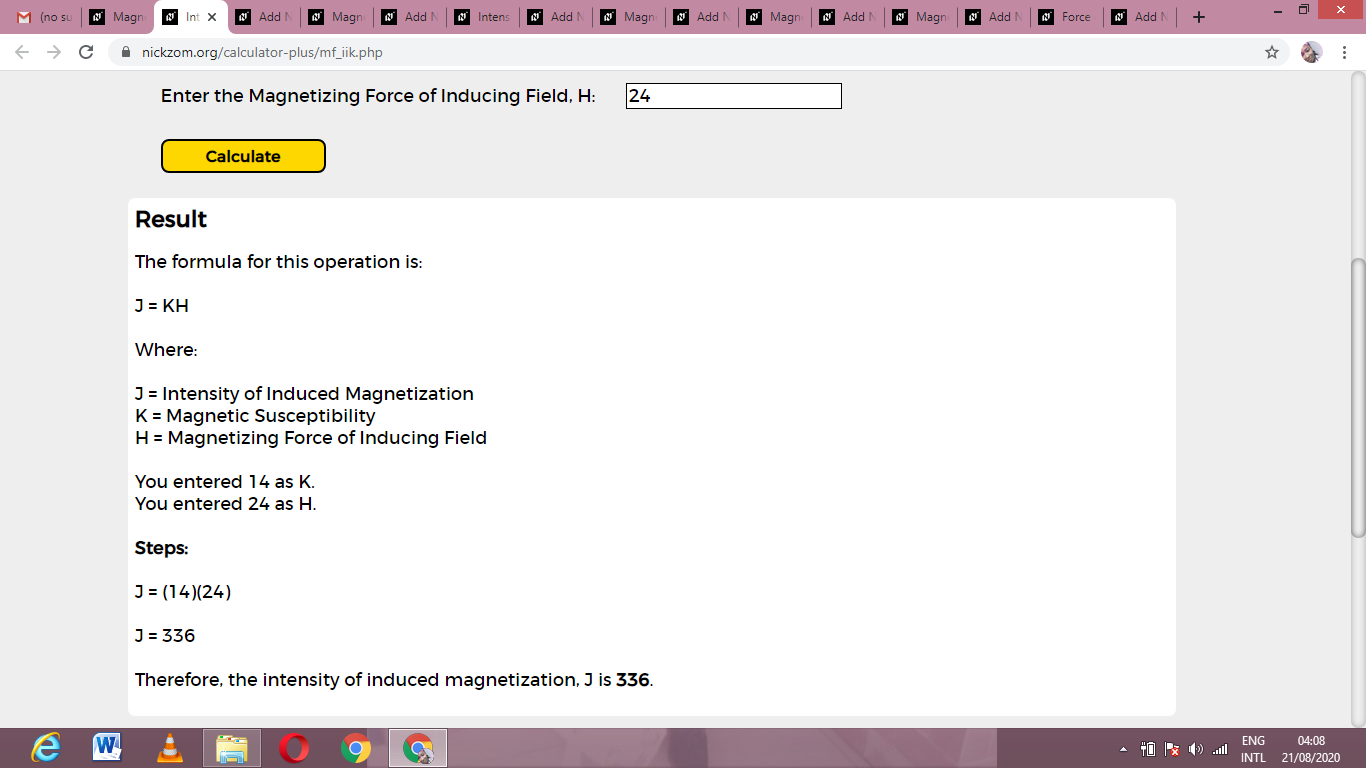# How to Calculate and Solve for Intensity of Induced Magnetization (K, H) | Magnetic FieldThe image above represents intensity of induced magnetization.

To compute for intensity of induced magnetization, two essential parameters are needed and these parameters are Magnetic Susceptibility (K) and Magnetizing Force of Inducing Field (H).

The formula for calculating intensity induced magnetization:

J = KH

Where:

J = Intensity of Induced Magnetization
K = Magnetic Susceptibility
H = Magnetizing Force of Inducing Field

Let’s solve an example;
Find the intensity induced magnetization when the magnetic susceptibility is 14 and the magnetizing force of inducing field is 24.

This implies that;

K = Magnetic Susceptibility = 14
H = Magnetizing Force of Inducing Field = 24

J = KH
J = (14)(24)
J = 336

Therefore, the intensity induced magnetization is 336.

Calculating the Magnetic Susceptibility when the Intensity Induced Magnetization and the Magnetizing Force of Induced Field is Given.

K = J / H

Where;

K = Magnetic Susceptibility
J = Intensity Induced Magnetization
H = Magnetizing force of Induced Field

Let’s solve an example;
Find the magnetic susceptibility when the intensity induced magnetization is 40 and the magnetizing force of Induced Field is 4.

This implies that;

J = Intensity Induced Magnetization = 40
H = Magnetizing force of Induced Field = 4

K = J / H
K = 40 / 4
K = 10

Therefore, the magnetic susceptibility is 10.

Calculating the Magnetizing Force for Induced Field when the Intensity Induced Magnetization and the Magnetic Susceptibility is Given.

H = J / K

Where;

H = Magnetizing Force for Induced Field
J = Intensity Induced Magnetization
K = Magnetic Susceptibility

Let’s solve an example;
Find the magnetizing force for induced field when the intensity induced magnetization is 35 and the magnetic susceptibility is 5.

This implies that;

J = Intensity Induced Magnetization = 35
K = Magnetic Susceptibility = 5

H = J / K
H = 35 / 5
H = 7

Therefore, the magnetizing force of induced field is 7.

Nickzom Calculator – The Calculator Encyclopedia is capable of calculating the intensity induced magnetization.

To get the answer and workings of the intensity induced magnetization using the Nickzom Calculator – The Calculator Encyclopedia. First, you need to obtain the app.

You can get this app via any of these means:

To get access to the professional version via web, you need to register and subscribe for NGN 2,000 per annum to have utter access to all functionalities.
You can also try the demo version via https://www.nickzom.org/calculator

Apple (Paid) – https://itunes.apple.com/us/app/nickzom-calculator/id1331162702?mt=8
Once, you have obtained the calculator encyclopedia app, proceed to the Calculator Map, then click on Geology under Add-on.Now, Click on Magnetic Field under GeologyNow, Click on Intensity Induced Magnetization under Magnetic FieldThe screenshot below displays the page or activity to enter your values, to get the answer for the intensity induced magnetization according to the respective parameters which are the Magnetic Susceptibility (K) and Magnetizing Force of Inducing Field (H).Now, enter the values appropriately and accordingly for the parameters as required by the Magnetic Susceptibility (K) is 14 and Magnetizing Force of Inducing Field (H) is 24.Finally, Click on CalculateAs you can see from the screenshot above, Nickzom Calculator– The Calculator Encyclopedia solves for the intensity induced magnetization and presents the formula, workings and steps too.